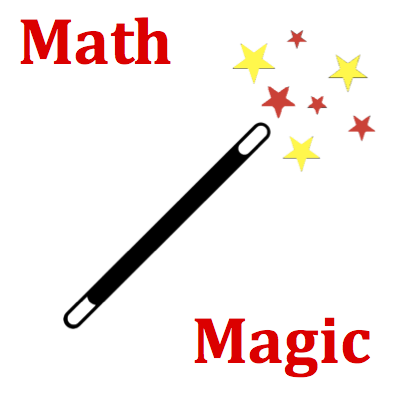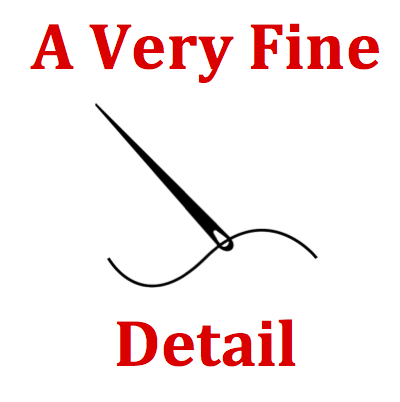# Reflection and Mirrors - Mission RM7 Detailed HelpIma Primpin places her 22.3-cm high face a distance of 24.9 cm from the surface of a concave mirror. If the mirror has a focal length of 33.9 cm, then what is the image distance and magnification of Ima's image?The mirror equation relates the object distance (do), the image distance (di) and the focal length (f):   1 / do + 1 / di = 1 / f The magnification equation relates the magnification (M), object height (ho), the image height (hi), object distance (do) and the image distance (di):   M = hi / ho = - di / do   The magnification value is an expression of the number of times bigger that the image height is than the object height.There are two unknowns to determine in this question - the image distance and the image magnification. Use the mirror equation to first determine the image distance. See the Math Magic section if necessary. The image distance will turn out to be a negative number, indicating that a virtual image is formed. Enter the value as a negative number.   Once calculated, use the magnification equation to determine the magnification. You will have to equate to the ratio of the image distance to object distance. There is a negative sign as part of the equation. See Formula Frenzy section.   It is important that you do not use rounded numbers in any calculation. Enter both answers to at least the second decimal place. See A Very Fine Detail section.The mirror equation, with its three reciprocal terms, is a little awkward to work with. It's recommended that you begin this problem by performing an algebra step to rearrange the equation such that the image distance (the unknown) is by itself on the left side of the equation. Then substitute the two numerical values into the equation and use your calculator to evaluate the right side. Once done, the reciprocal of the image distance is equal to the number on your calculator. Take the reciprocal of this number to determine the image distance.The Minds On Physics program calculates answers accurate to the fourth decimal place. Your answer does not need to be that accurate. The program will allow you to have a 1 percent deviation from the right answer without being wrong. This means two things: 1) Enter your answer with at least three significant digits. 2) Do not round any numbers until your final calculation.